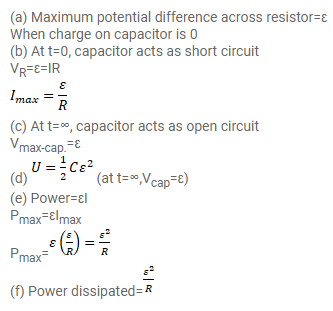# A capacitance C, a resistance R and

Question:

A capacitance $C$, a resistance $R$ and an emf $\varepsilon$ are connected in series at $t=0$. What is the maximum value of,

(a) The potential difference across the resistor,

(b) The current in the circuit,

(c) The potential difference across the capacitor,

(d) The energy stored in the capacitor,

(e) The power delivered by the battery and

(f) The power converted into heat.

Solution: